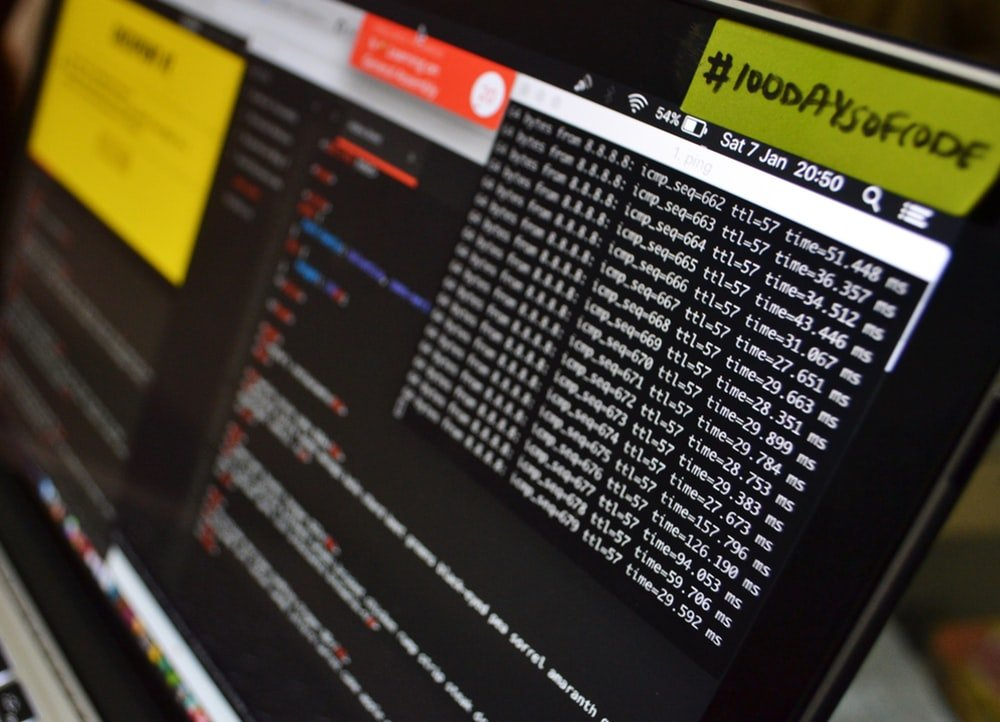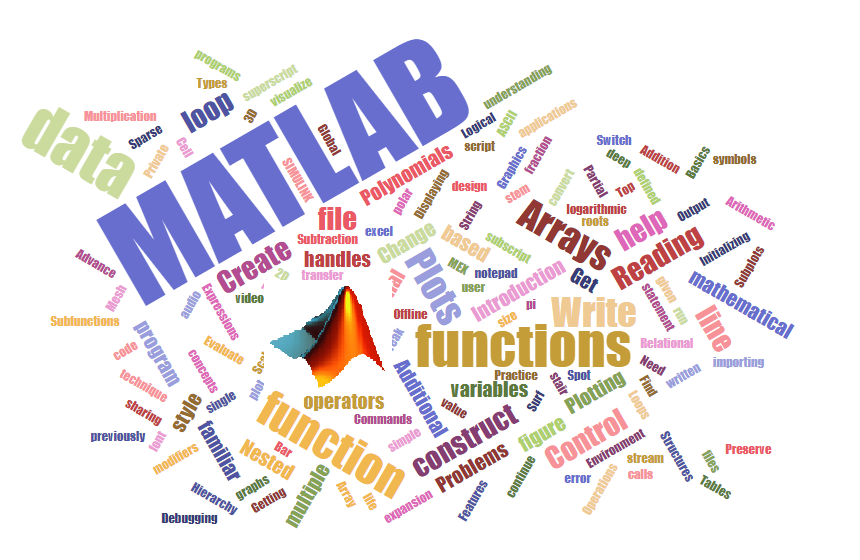# MATLAB Programming

## MATLAB ProgrammingMATLAB is a platform for the researchers to implement and analyze their research problems. Now days MATLAB has become the programming language of every engineer. If you are doing masters or doctorate, definitely you have to use this software because its capabilities are amazing. This course is designed for you, to provide basic knowledge of MATLAB and give a start to your project.

## course ModulesObjective: After doing this module you will be much confident about MATLAB Programming and able to write MATLAB Code for a given problem, understand the previous written code and debug yours or others  MATLAB codes.

Syllabus: MATLAB Programming Concepts

MATLAB Basics:

Introduction to MATLAB, Get familiar with MATLAB Environment, Get familiar with MATLAB Commands, How to write Mathematical Expressions in MATLAB, How to write a simple MATLAB Program, Variables and Arrays, Multidimensional arrays, Initializing variables in MATLAB, Reading data from notepad and excel file, reading data from audio and video files, Reading and visualize image data, Displaying Output data, Scalar & Array Operations.

Plotting:

Introduction to Plotting, Multiple Plots: Subplots, Additional 2D plots: logarithmic, Bar, stem, stair, pi, polar, histogram, compass; 3D Plots: Mesh, Surf, waterfall, contour, patch, cone, sphere etc.; Additional Plotting Features: Axis control, plot multiple graphs in a single figure, Change the line color and style, Change font style and size, Write subscript and superscript, Write mathematical symbols in MATLAB figure, stream modifiers.

Loops and Control Statements:

Top down design technique, Need of Control statement, Operators: Arithmetic, Relational and Logical, Hierarchy of operators; if construct, Nested if construct, Switch construct, try and catch construct, While loop, for loop, Break and continue, loop based problems.

Functions:

How to create a user defined function, convert MATLAB script to a function, Types of functions: Sub-functions, Nested functions, Private functions, Global data sharing, Preserve data between calls, String Functions.

Cell Arrays, Sparse Arrays, Structures, Function handles, Graphics handles, data importing through functions, Tables. live scripting and publishing of formatted codes and results in pdf.

Numerical Computation Techniques:

Polynomial handling, Symbolic mathematics toolbox, Integration, Differentiation, Laplace transforms, Ordinary Differential Equations: Euler’s method, Ranga Kutta method, Non-linear equations Newton-Raphson method, Interpolation, curve fitting toolbox.

Debugging:

Spot an error in previously written code, deep understanding of the MATLAB programs, run program line by line.

Getting MATLAB help

Objective: After doing this module you will be able to write MATLAB Code for a given problem, understand the previous written code, debug the MATLAB code apart from that you can create your own standalone application that can run without MATLAB on any system.

## Syllabus for the Module:

MATLAB Programming Concepts [Basic Module] complete syllabus+

Graphical User Interface (GUI): Introduction and application of GUI, Get familiar with GUI working Environment, Planning and Designing layout for GUI App, GUI component Programming for – PUSH Buttons, Toggle Button, Static and Edit text box, Checkbox and Radio Buttons, Slider, Axes, Popup menu, Panels, Button Group, Dialog boxes, Passing data through different callback functions, Create Your own MATLAB APP.

Coming soon…